﻿ 潜艇圆形流水孔的溢流特性分析
 舰船科学技术2022, Vol. 44Issue (14): 12-17    DOI: 10.3404/j.issn.1672-7649.2022.14.003PDF

1. 海军工程大学 舰船与海洋学院，湖北 武汉 430033;
2. 海军研究院，北京 100071

Analysis of overflow characteristics of circular water hole of submarine
WEI Ke-ke1, GAO Xiao-peng1, MA Cheng2
1. Ship and Ocean College, Naval Engineering University, Wuhan 430033, China;
2. Naval Academy, Beijing 100071, China
Abstract: In order to explore and study the effect of different sizes of water holes on the backwater and water outflow from the submarine’s superstructure, based on the calculation method of sliding grid and with interaction between internal and external domains. Taking the local hull model with superstructure water holes as the research object, numerical simulation of the overflow of circle water holes of different scales at different draughts was carried out, and the superstructure residuals of circle water holes of different sizes were compared and analyzed. The change law of parameters such as water volume, residual water height, orifice flow and discharge coefficient. The research results show that: the smaller the shrinkage of the water hole, the larger the size of the water hole, the faster the change in the volume and height of the remaining water in the non-watertight superstructure, and the greater the flow of the water hole. Through comprehensive comparison and analysis, the flow coefficient of the flow hole with the reduction ratio1:16.67 is the best. The calculation method and the related conclusions can provide some theoretical support for the design of water hole of real submarine.
Key words: circle water hole     interaction between internal and external domains     flow coefficient
0 引　言

1 计算方法 1.1 控制方程

 $\left\{ \begin{gathered} \frac{{\partial {{\bar u}_i}}}{{\partial t}} + {{\bar u}_j}\frac{{\partial {{\bar u}_i}}}{{\partial {x_j}}} = - \frac{1}{\rho }\frac{{\partial \bar p}}{{\partial {x_i}}} + \frac{1}{\rho }\frac{\partial }{{\partial {x_j}}}\left(\mu \frac{{\partial {{\bar u}_i}}}{{\partial {x_j}}} - \rho \overline {{{u'}_i}{{u'}_j}} \right) + {f_i}，\\ \frac{{\partial {{\bar u}_i}}}{{\partial {x_i}}} = 0 。\\ \end{gathered} \right.$ (1)

 $\frac{{{\rm{d}}\chi }}{{{\rm{d}}t}} = \frac{{3 \times \left[ {\chi (n + 1) - \chi (n)} \right] - \left[ {\chi (n) - \chi (n - 1)} \right]}}{{2 \times \Delta t}}。$ (2)

1.2 流水孔溢流参数方程

 $\left\{ \begin{gathered} {V_{{\rm{water}}}} = \int {a{\rm{d}}V}，\\ {Q_{{\rm{mass}}}} = \int {Q{\rm{d}}S/} {S_0}，\\ {H_{{\rm{average}}}} = \int H {\rm{d}}S/{S_{iso}}。\\ \end{gathered} \right.$ (3)

 $\mu = \frac{{{Q_{{\rm{mass}}}}}}{{\rho {S_0}\sqrt {2g{H_{{\rm{average}}}}} }}，$ (4)
 $H' = {H_{{\rm{average}}}}/D。$ (5)
1.3 内外域交互的计算方法及计算流程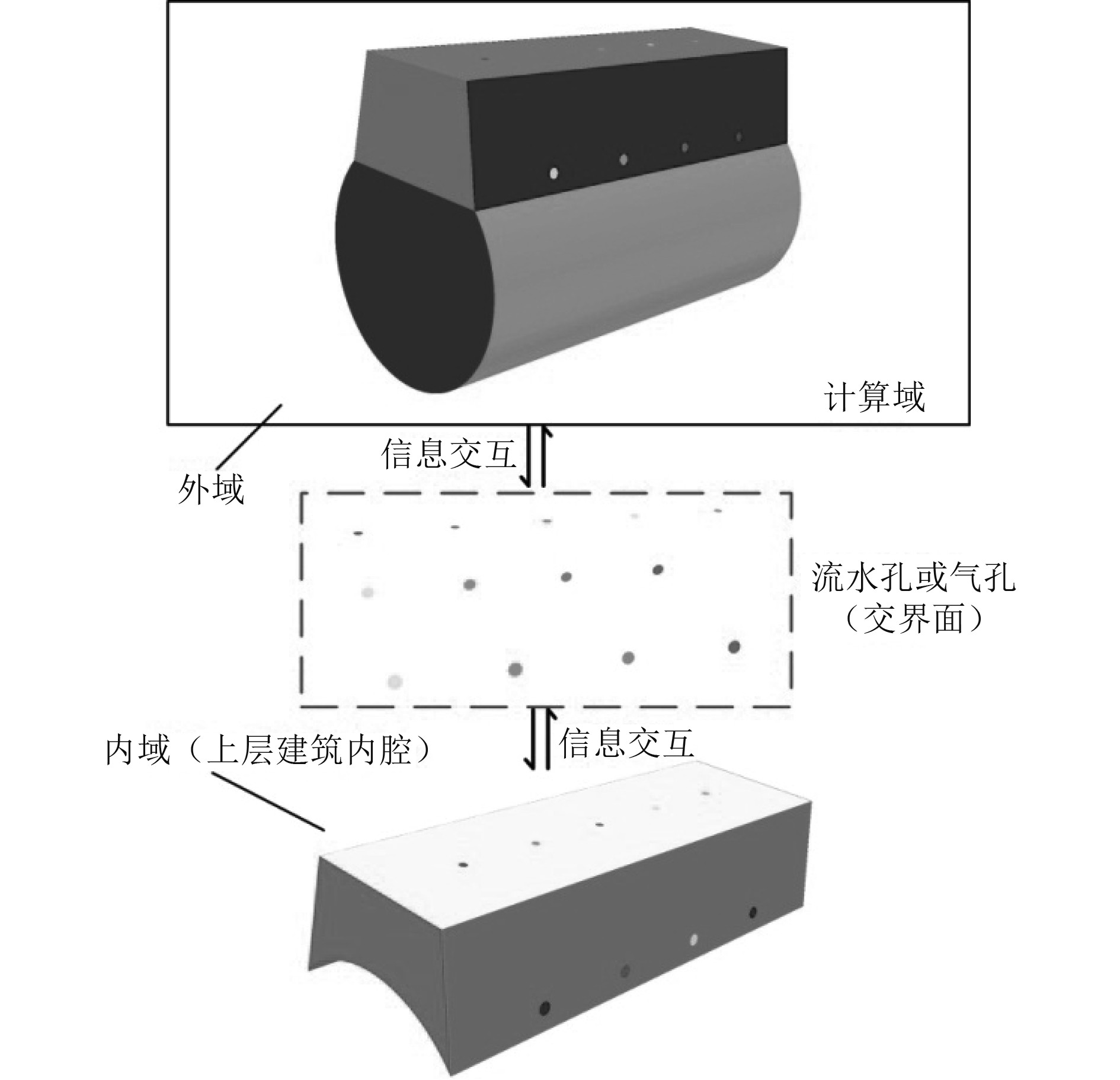图 1 内域与外域交互的示意图 Fig. 1 Schematic diagram of the interaction between inner and outer domains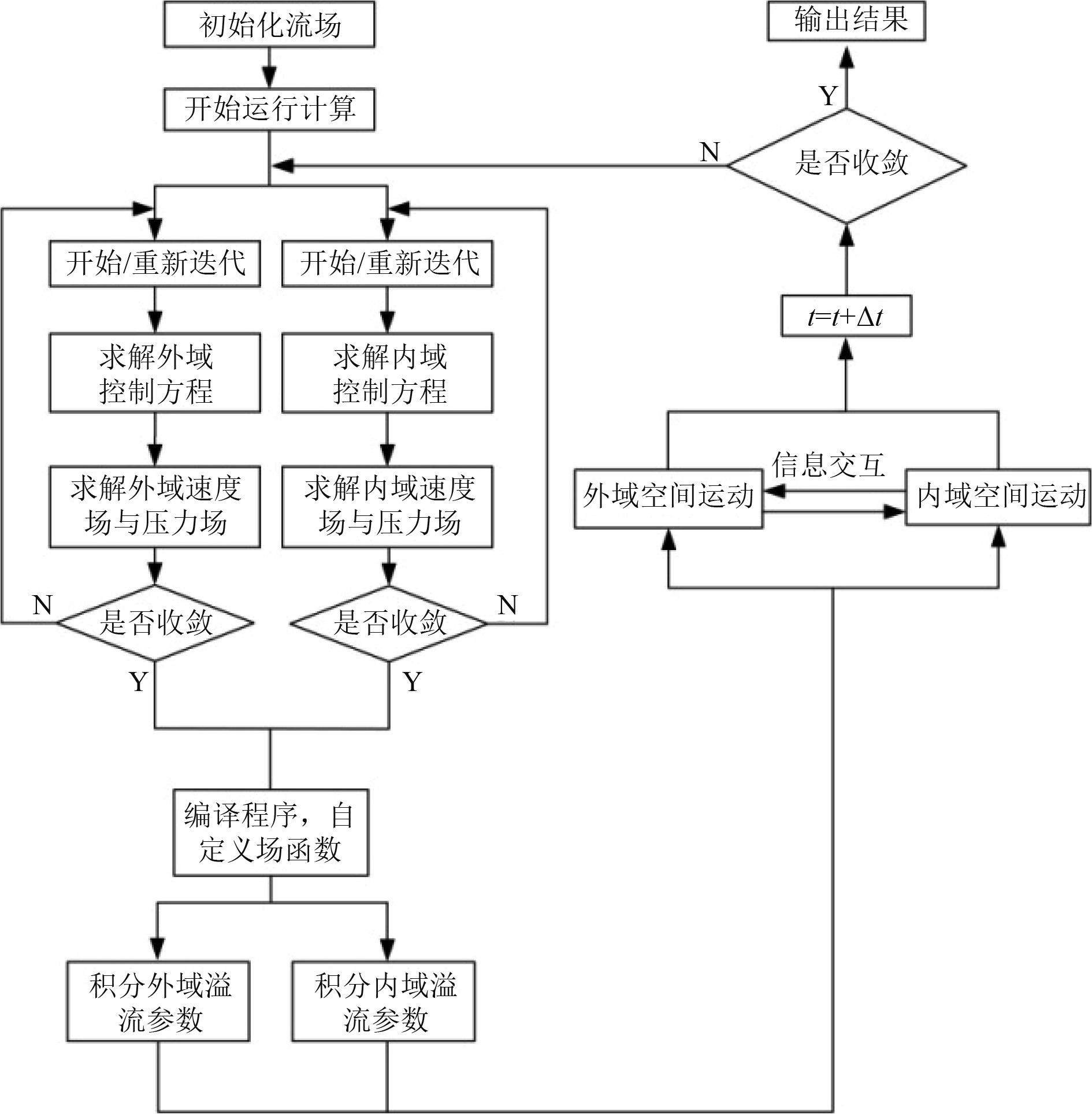图 2 内域与外域交互计算方法的流程图 Fig. 2 Flowchart of computation methods for the interaction between inner and outer domains
2 计算对象及计算工况 2.1 运动模型表 1 模型主尺度参数 Tab.1 Main scale parameters of the model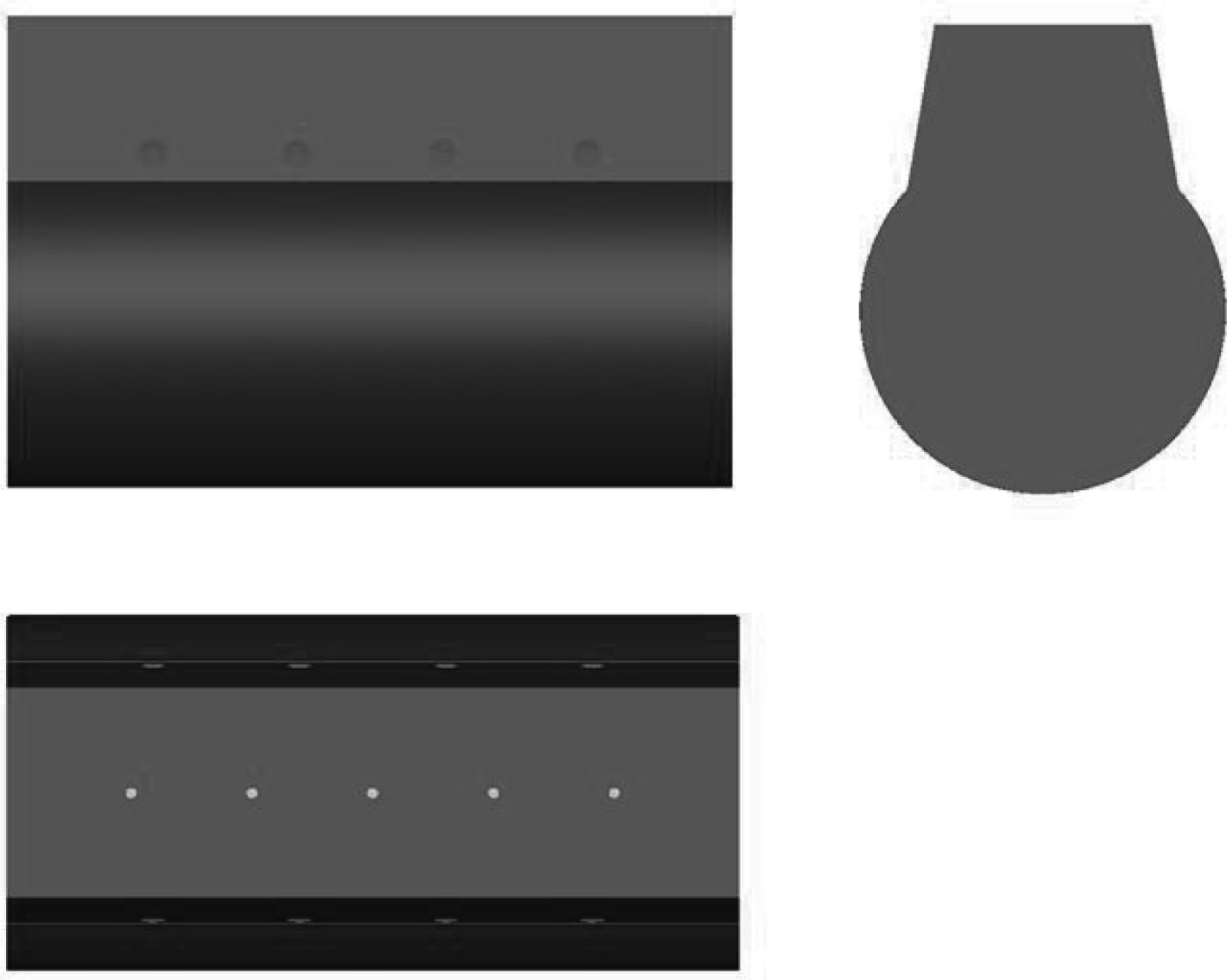图 3 含上建流水孔的艇中局部模型 Fig. 3 A partial model of the middle part of submarine
2.2 计算域及网格划分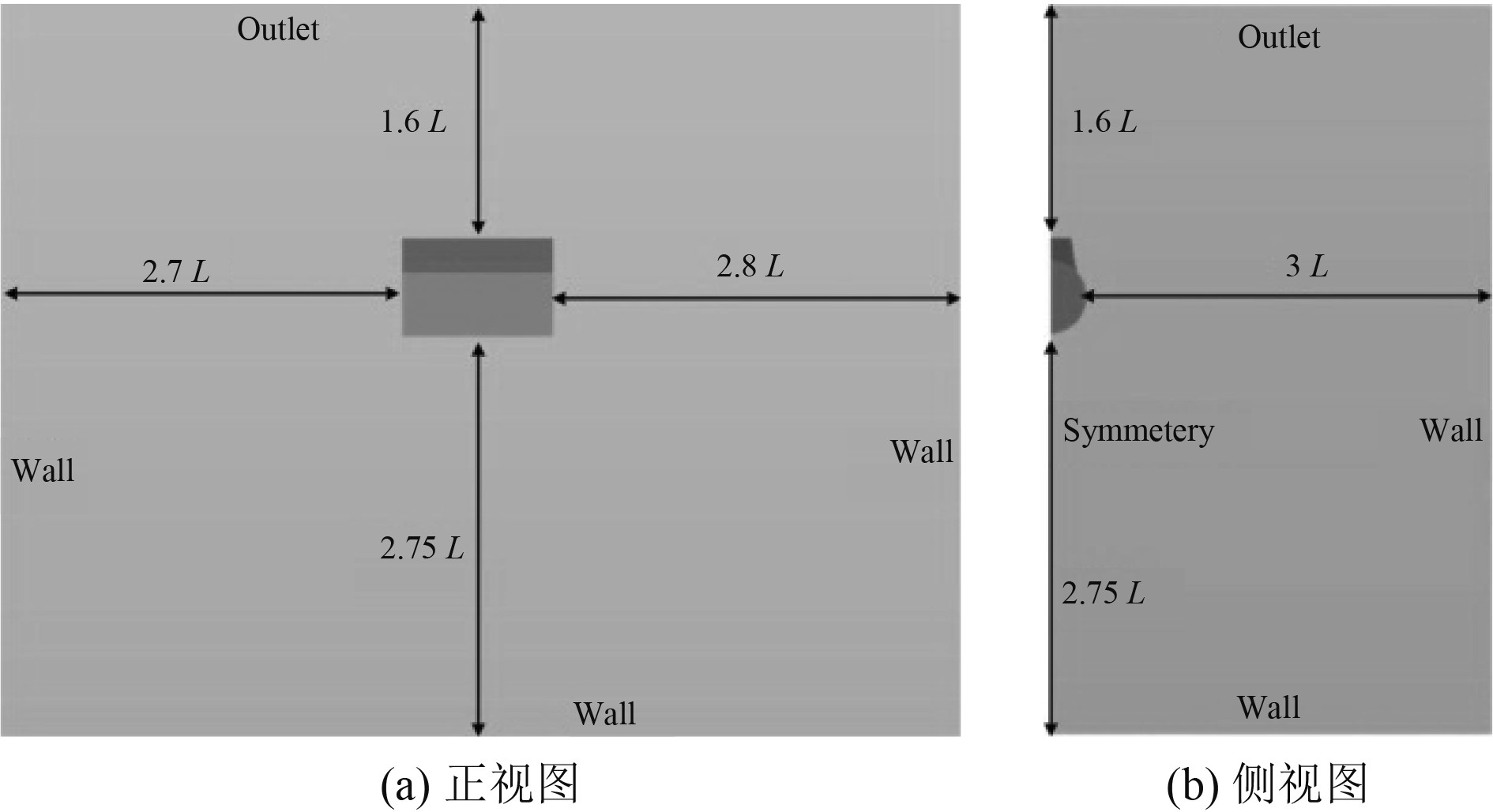图 4 计算域及边界类型 Fig. 4 Calculate the domain and boundary conditions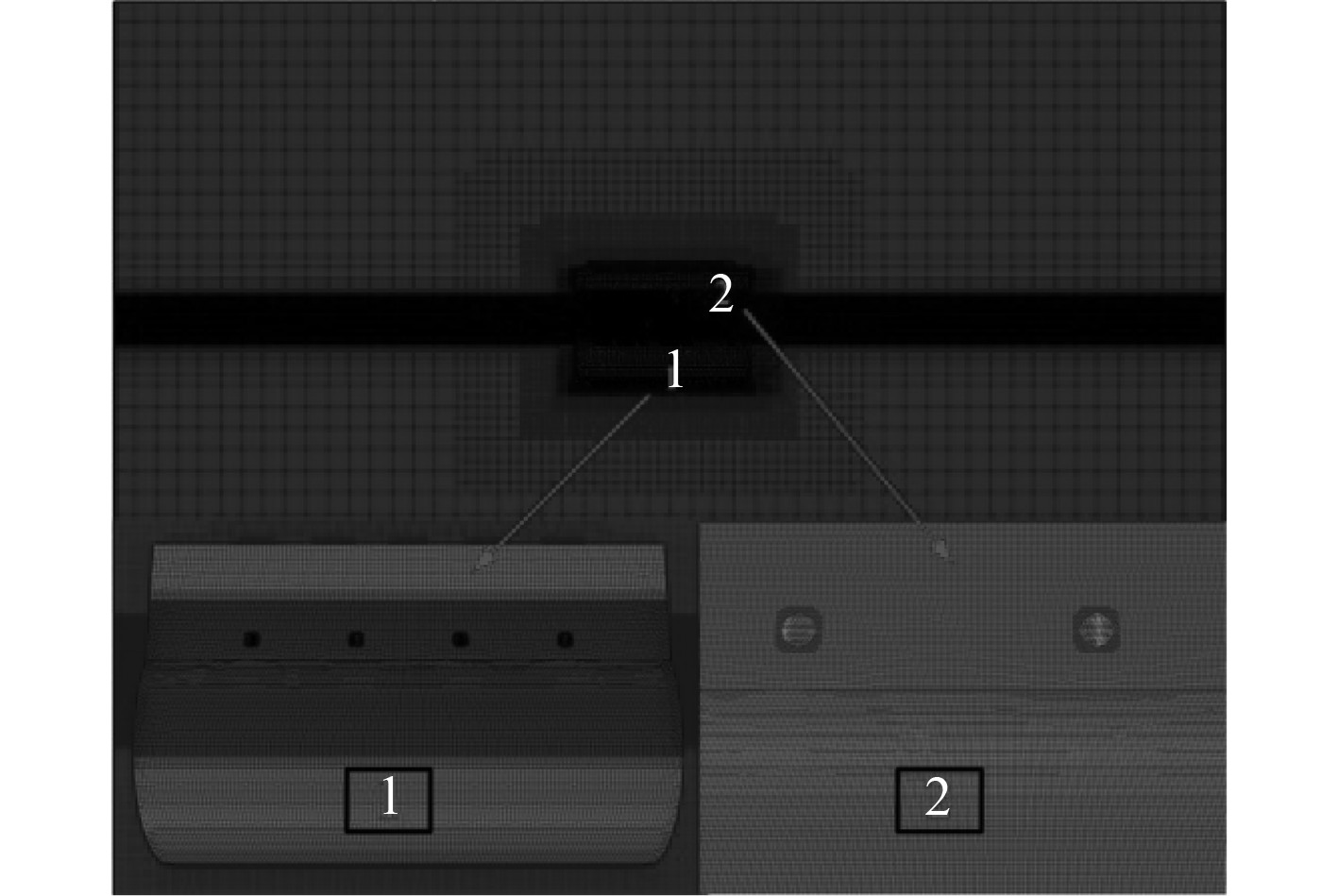图 5 外域网格 Fig. 5 Outland grid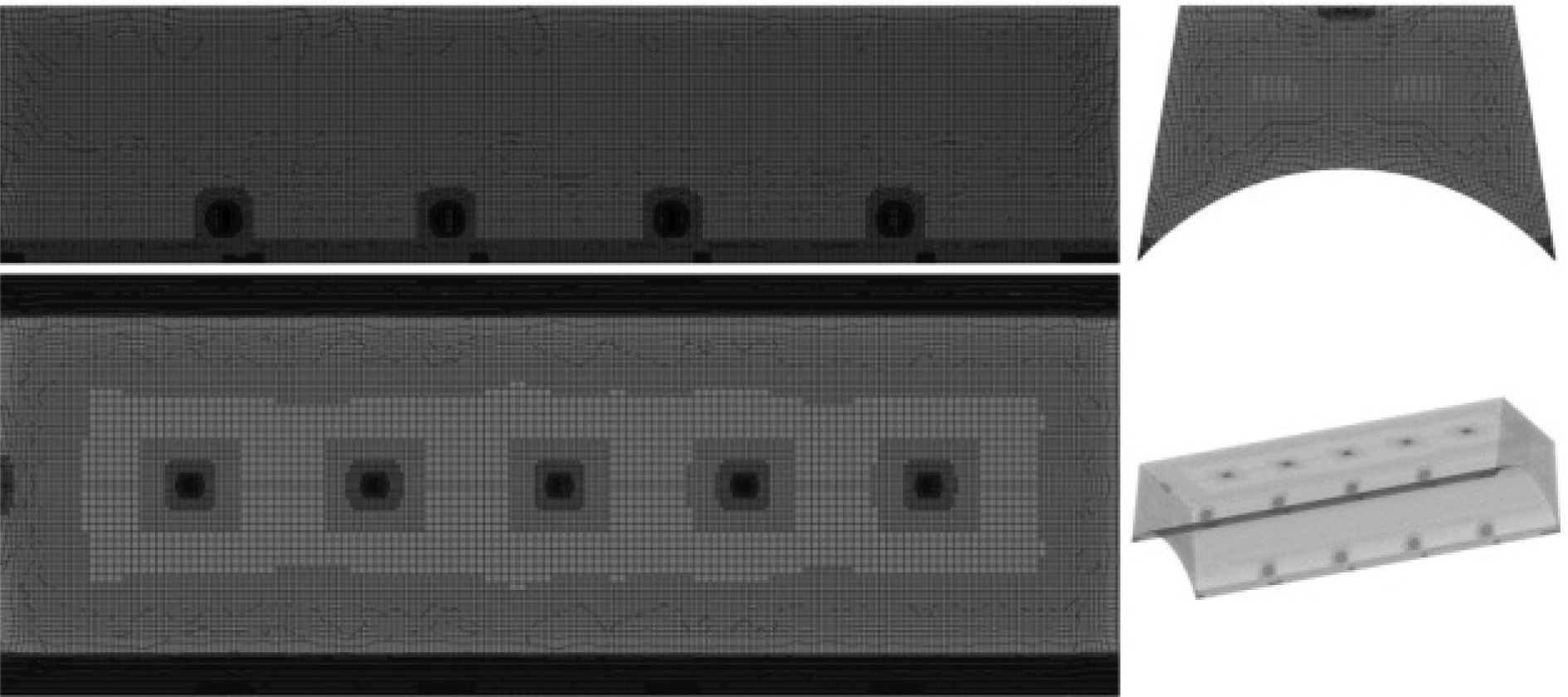图 6 内域网格 Fig. 6 Domain grid
2.3 计算工况表 2 流水孔的形式及尺寸大小 Tab.2 Form and size of water hole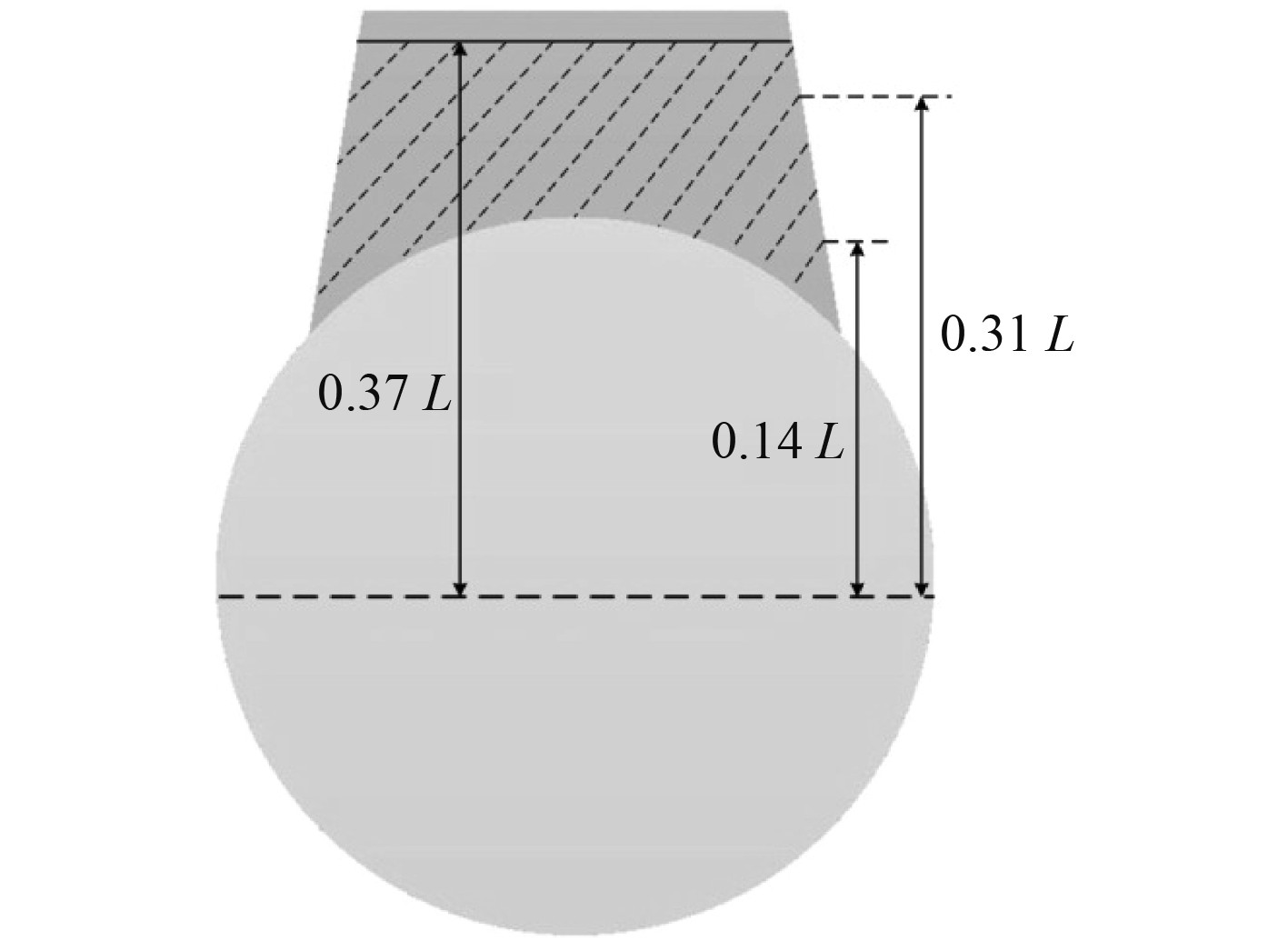图 7 模型的吃水示意图 Fig. 7 The draft diagram of the model
3 计算结果及分析 3.1 流水孔溢流的流场分析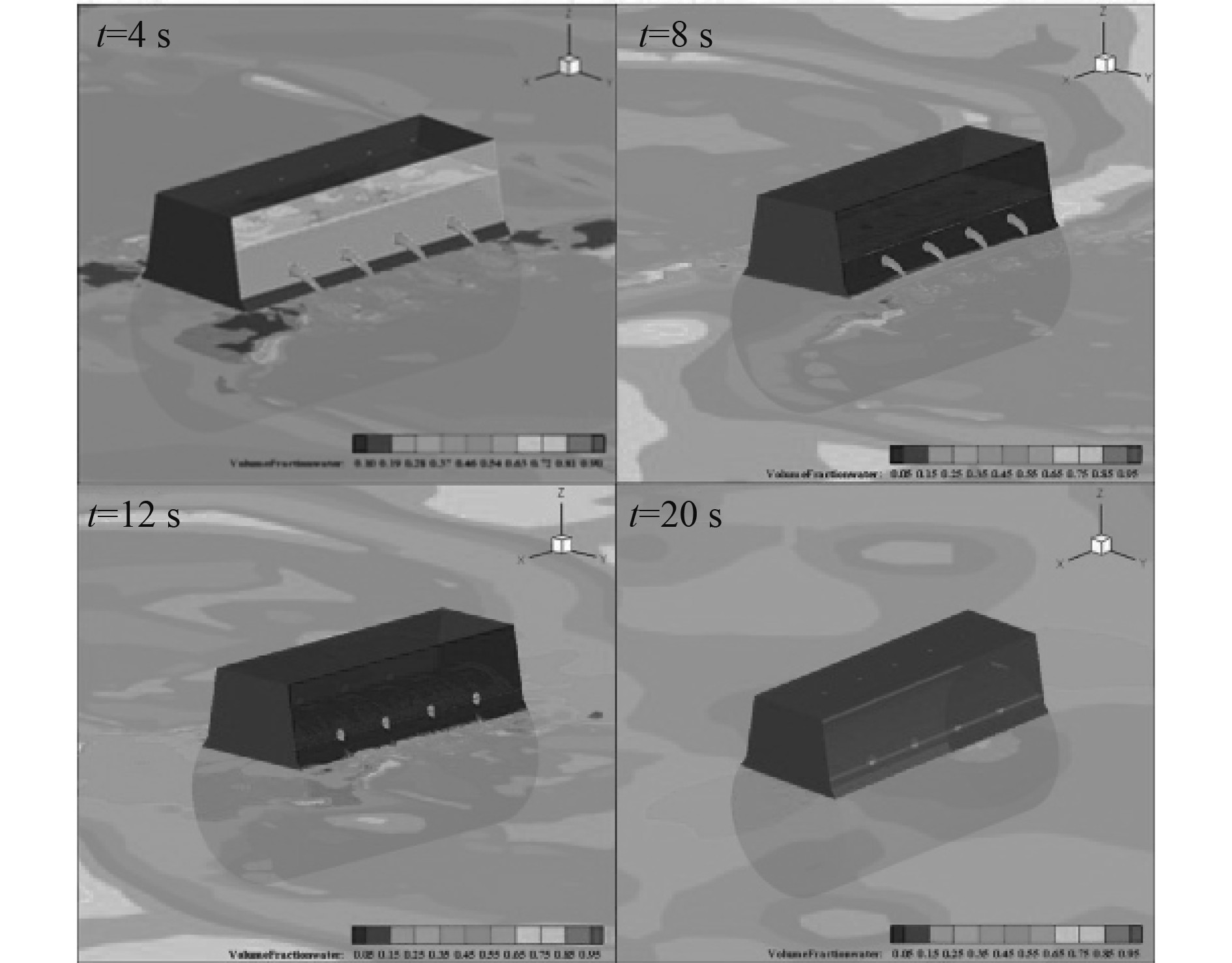图 9 缩比为1∶16.67圆形流水孔的溢流情况 Fig. 9 The overflow condition of circle water hole with scale is 1∶16.67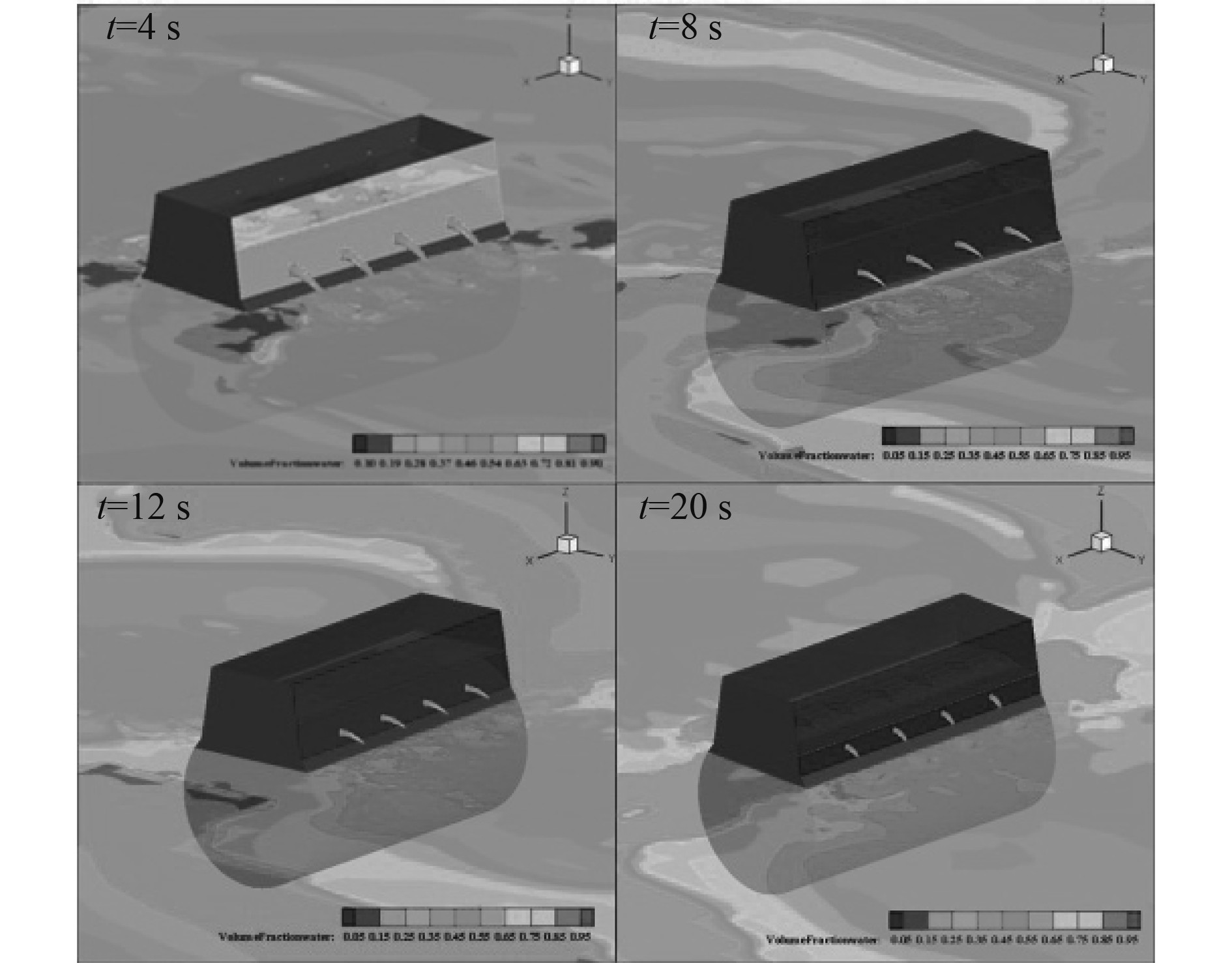图 8 缩比为1∶25圆形流水孔的溢流情况 Fig. 8 The overflow condition of circle water hole with scale is 1∶25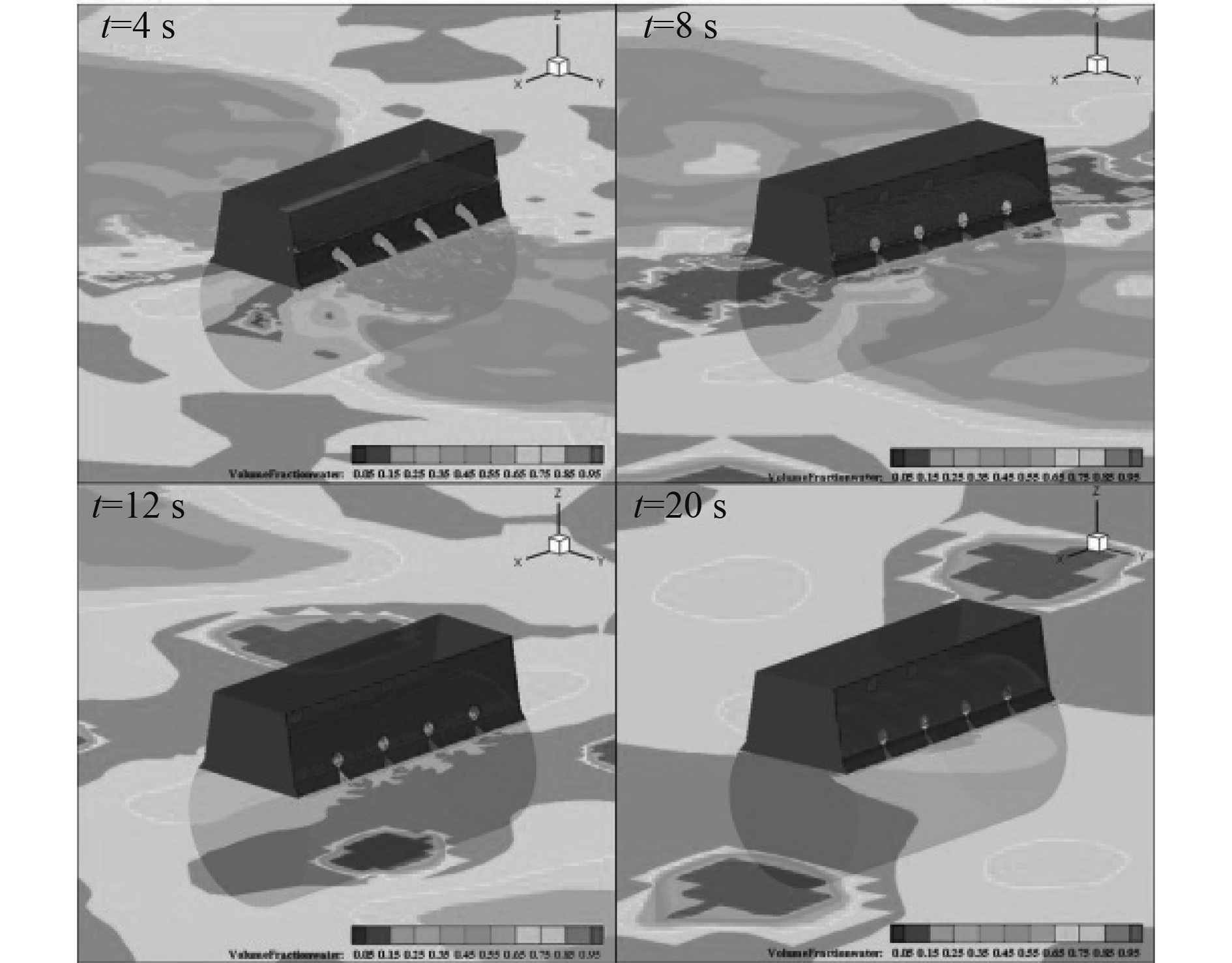图 10 缩比为1∶12.5圆形流水孔的溢流情况 Fig. 10 The overflow condition of circle water hole with scale is 1∶12.5
3.2 计算结果及分析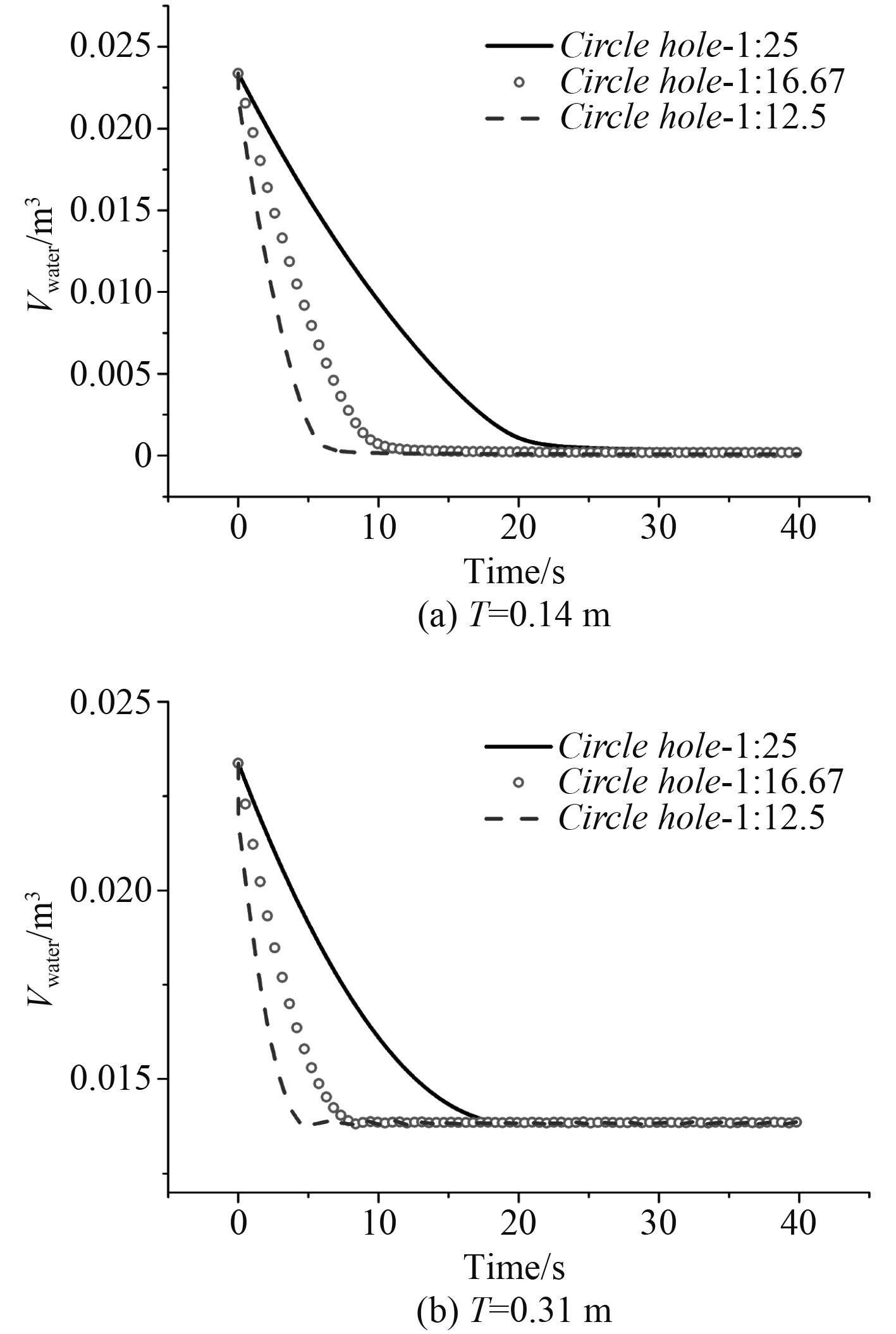图 11 不同圆形流水孔的上层建筑余水容积的时历曲线 Fig. 11 Time series of residual water volume of superstructure with different circle water holes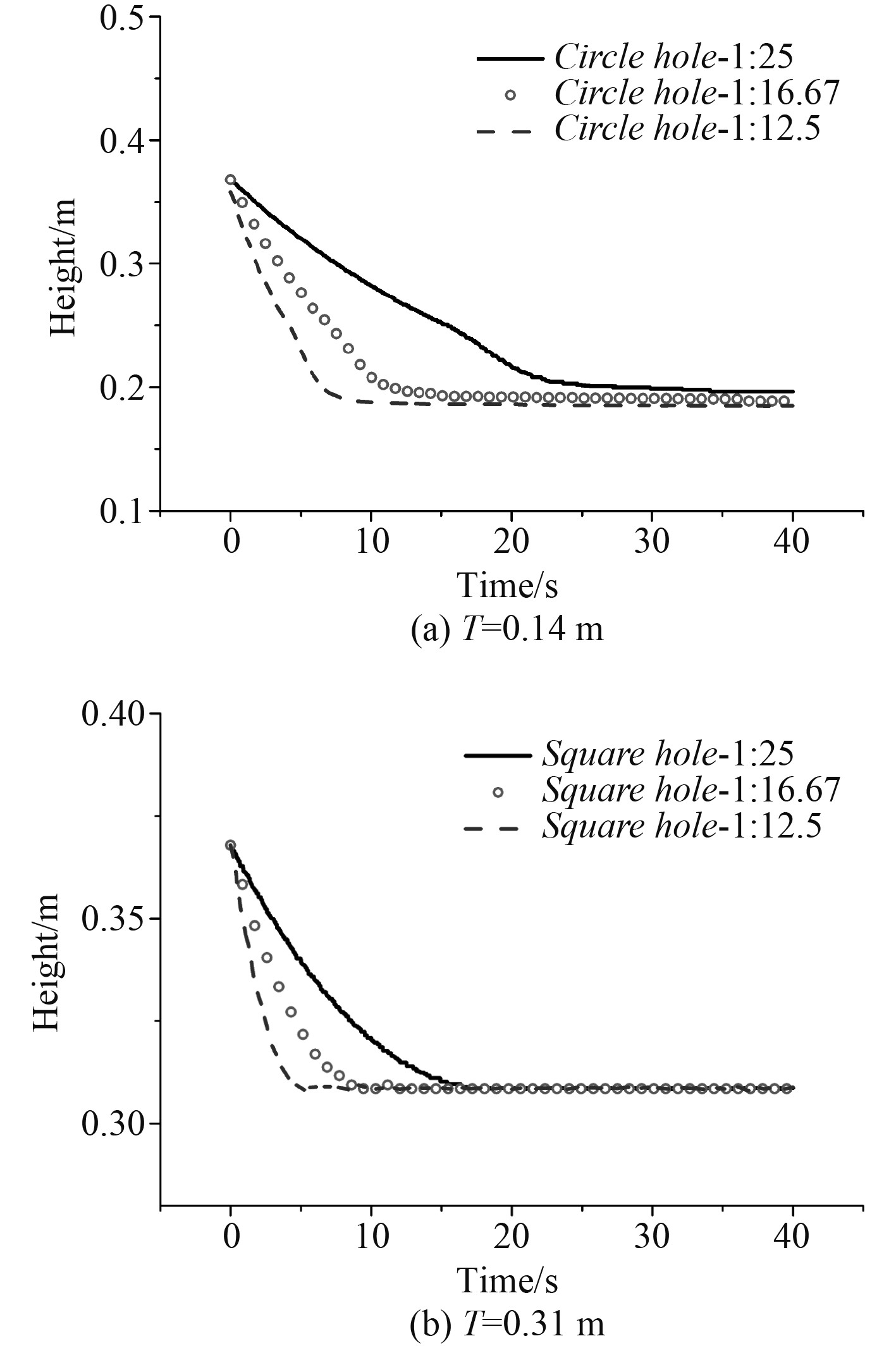图 13 不同圆形流水孔上层建筑余水的高度的时历曲线 Fig. 13 Time series of residual water height of superstructure with different circle water holes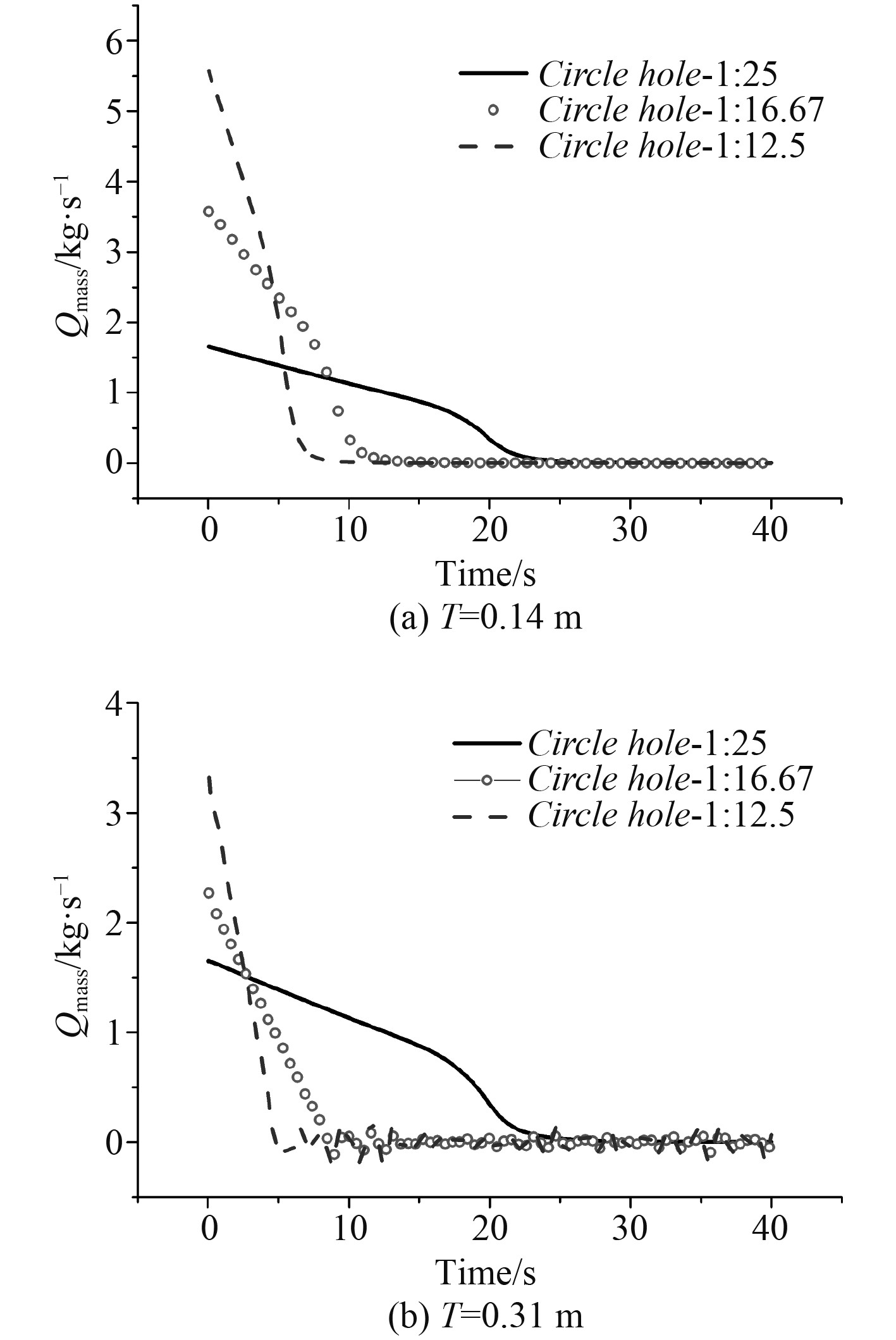图 12 不同圆形流水孔的流量时历曲线 Fig. 12 Time series of the rate of flow with different circle water holes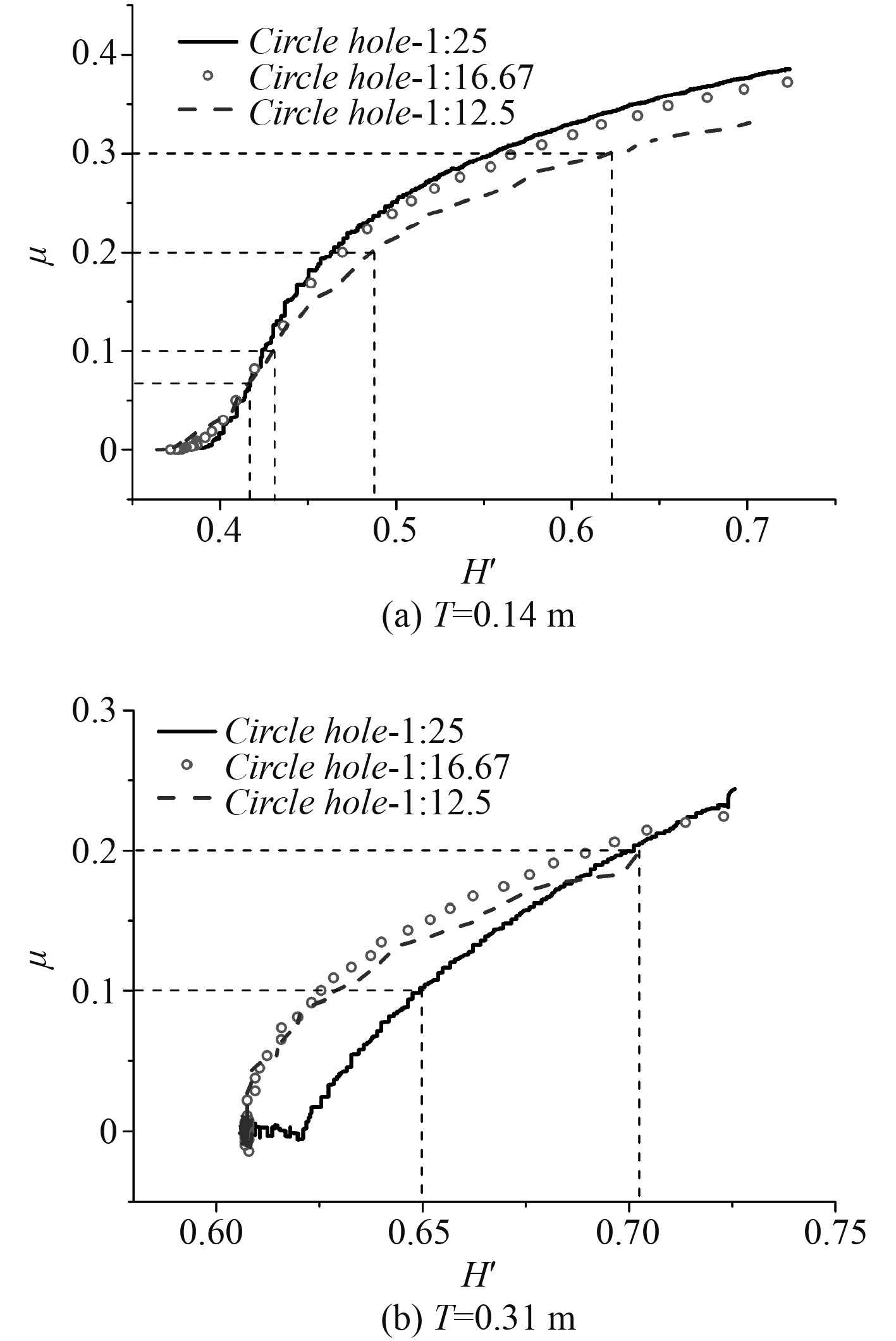图 14 不同圆形流水孔的流量系数 Fig. 14 Flow coefficient of different square water holes

4 结　语

1）基于滑移网格，采用内外域交互的计算方法，对潜艇的局部含上层建筑模型在不同吃水下不同尺寸大小流水孔的流量系数开展数值计算，该方法可为流水孔的特性分析和实艇的流水孔方案设计提供一定的借鉴。

2）通过对比分析不同缩比的圆形流水孔在不同吃水下流量系数随无因次高度差的变化，研究发现流水孔的尺寸越大，其非水密上层建筑内余水的容积和余水的高度变化值越快，流水孔的流量越大；综合对比分析，圆形流水孔缩比为1∶16.67的流量系数最优，因此，实艇流水孔尺寸大小及总面积的优化设计中，根据换算可参考此缩比的流水孔尺寸进行优化设计。

  孟生, 张宇文, 裴環. 潜艇流水孔阻力特性分析[J]. 计算机测量与控制, 2011, 19(5): 1098-1011.  孟生, 张宇文, 李林杰. 潜艇流水孔偏角特性研究[J]. 计算机仿真, 2011, 28(5): 17-25. DOI:10.3969/j.issn.1006-9348.2011.05.005  张航, 冯大奎, 蔡潇, 等. 开孔分布对空腔流动特性的分析[J]. 舰船科学技术, 2017, 39(6): 48-52. DOI:10.3404/j.issn.1672-7619.2017.06.010  张楠, 沈泓萃, 姚惠之. 带流水孔潜体流场数值模拟[J]. 船舶力学, 2004, 8(1): 1-11. DOI:10.3969/j.issn.1007-7294.2004.01.001  张楠, 沈泓萃, 姚惠之. 潜艇流水孔阻力数值计算与回归分析研究[J]. 船舶力学, 2004, 8(4): 5-15. DOI:10.3969/j.issn.1007-7294.2004.04.002  张言才, 宋建荣. 船舶压载水舱流水孔选型的模糊综合评判[J]. 江苏船舶, 2014, 31(3): 4-7. DOI:10.3969/j.issn.1001-5388.2014.03.002  张允. 开孔潜体流场与声场的数值模拟[D]. 上海: 上海交通大学, 2011.  熊鳌魁, 仲夏, 包胜平. 二维潜艇流水孔空腔减阻数值研究[J]. 武汉理工大学学报(交通科学与工程版), 2014, 38(2): 280-284.  李维嘉, 江涛, 蔡斌. 基于ADAMS优化设计的某潜艇用流水孔启闭装置[J]. 中国舰船研究, 2014, 9(4): 113-119. DOI:10.3969/j.issn.1673-3185.2014.04.019